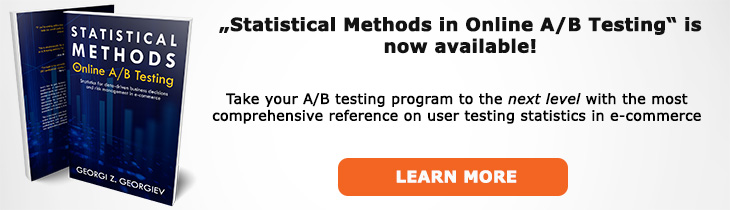# What does "Proportion" mean?

Definition of Proportion in the context of A/B testing (online controlled experiments).

## What is a Proportion?

Aliases: rate

A proportion, a.k.a. rate in A/B testing usually refers to the frequency of occurrence of an event in a sample or population, for example the presence or absence of a conversion event for users in a particular sample. Other notable examples include conversion rate, purchase rate, churn rate, bounce rate, drop-off rate, etc.

A proportion is calculated as the sum x1, x2, ... , xn divided by n, which, given that the possible values are only zero or one (proportions and rates are used for binomial metrics) can be simplified to N1/N0 where N1 is the number of events and N0 is the number of possible events that did not occur, e.g. users that did not convert.

In many A/B tests we compute statistics related to proportions which are often converted to percentages for ease of interpretation and presentation. Most-often we care about measuring the uncertainty around an observed percent change and more rarely about an observed absolute difference.

A proportion, being an arithmetic mean, has properties all properties of the mean is thus a good sample statistic: it is an asymptotically consistent estimator, as well as being a finite-sample unbiased estimator, fully-efficient estimator and a sufficient estimator. In short, as sample size increases the sample proportion consistently approaches the population proportion and for any finite number of samples n the proportion does not show systematic bias, there is no other estimator that results in faster tests with the same accuracy and there is no other statistic that can be calculated from the same sample that provides any additional information as to the value of the parameter. All of the above properties are transferred to any frequentist hypothesis test or estimation procedure (e.g. significance test, confidence interval or Maximum Likelihood Estimate) that makes use of proportions.

A proportion which is near one of the extremes (0 or 1) might shift significantly due to only a few observations on the other end of the parameter space. The proportion is guaranteed to not coincide with any actual value present in the sample unless it is exactly 0 or exactly 1 since they are all either 0 or 1.

Like this glossary entry? For an in-depth and comprehensive reading on A/B testing stats, check out the book "Statistical Methods in Online A/B Testing" by the author of this glossary, Georgi Georgiev.## Glossary Index by Letter

Select a letter to see all A/B testing terms starting with that letter or visit the Glossary homepage to see all.